# 一类含脉冲项的Gronwall型积分不等式的推广Generalization of Integral Inequalities of the Gronwall Type for Discontinuous Function

• 全文下载: PDF(432KB)    PP.83-88   DOI: 10.12677/PM.2019.91011
• 下载量: 329  浏览量: 476   科研立项经费支持

This paper investigates generalization of integral inequalities of Gronwall type for discontinuous function by using the mathematical induction. It generalizes the existing forms and the results are more universal.

1. 引言

Gronwall型积分不等式和它们的各种推广形式在讨论微分方程解的存在性、唯一性、连续性、有界性、稳定性和不变流形等方面有着十分重要的作用。关于连续函数的积分不等式及其应用方面已有很多结果，如文献     等。

$u\left(t\right)\le c+{\int }_{{t}_{0}}^{t}f\left(s\right)u\left(s\right)\text{d}s+\underset{{t}_{0}<{t}_{i}(1)

2005年，Samoilenko和Perestyuk  用单调不减函数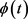代替常数讨论了如下Bellman-Bihari型积分不等式

$u\left(t\right)\le \varphi \left(t\right)+{\int }_{{t}_{0}}^{t}f\left(s\right){u}^{m}\left(s\right)\text{d}s+\underset{{t}_{0}<{t}_{i}0$(2)

2007年，Iovane  又研究了含时滞项 $p\left(t\right)$ 的非连续函数积分不等式

$u\left(t\right)\le \varphi \left(t\right)+{\int }_{{t}_{0}}^{t}f\left(s\right){u}^{m}\left(p\left(s\right)\right)\text{d}s+\underset{{t}_{0}<{t}_{i}0$(3)

$u\left(t\right)\le \varphi \left(t\right)+g\left(t\right){\int }_{{t}_{0}}^{t}f\left(s\right)\omega \left(u\left(p\left(s\right)\right)\right)\text{d}s+\underset{{t}_{0}<{t}_{i}(4)

$\varphi \left(u\left(t\right)\right)\le {c}_{0}+\underset{i=1}{\overset{n}{\sum }}{\int }_{{\alpha }_{i}\left({t}_{0}\right)}^{{\alpha }_{i}\left(t\right)}{u}^{q}\left(s\right)\left[{f}_{i}\left(s\right)\omega \left(u\left(s\right)\right)+{g}_{i}\left(s\right)\right]\text{d}s+h\left(t\right)\underset{{t}_{0}<{t}_{j}(5)

2. 主要结果

(H1) $\varphi \left(t\right)$ 是定义在 $\left[\text{0},\infty \right)$ 的非负单增连续函数且 $\varphi \left(\infty \right)=\infty$

(H2)是定义在 $\left[{t}_{0},\infty \right)$ 上的非负连续函数， ${\alpha }_{i}\left(t\right)$ 是定义在的非负可导函数，且 ${\alpha }_{i}\left(t\right)\le t，i=1,2,\cdots ,n$$t\in \left[{t}_{i-1},{t}_{i}\right]$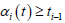(H3)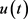是定义在 $\left[{t}_{0},\infty \right)$ 上的非负分段连续函数，其中 $0\le {t}_{0}<{t}_{1}<\cdots$${t}_{j}$$u\left(t\right)$ 的第一类不连续点， $u\left({t}_{{}_{j}}-0\right)=\underset{t\to {t}_{j}{}^{-}}{\mathrm{lim}}u\left(t\right)$$\underset{j\to \infty }{\mathrm{lim}}{t}_{j}=\infty$

(H4) $\omega \left(u\right)$ 是定义在 $\left[\text{0},\infty \right)$ 上的单调不减连续函数，且当 $u>0$ 时， $\omega \left(u\right)>0$

(H5) ${c}_{0},q,m,{\beta }_{j}$ 都是常数且 ${c}_{0}\ge \text{0},q>0,m>0,{\beta }_{j}\ge 0,j=1,2,\cdots$

${u}_{j}\left(t\right)\le {\varphi }^{-1}\left\{{G}^{-1}\left[{W}^{-1}\left(W\left({p}_{j}\left(t\right)\right)+\underset{i=1}{\overset{n}{\sum }}{\int }_{{\alpha }_{i}\left({t}_{j}\right)}^{{\alpha }_{i}\left(t\right)}{f}_{i}\left(s\right)\text{d}s\right)\right]\right\},t\in \left[{t}_{j},{t}_{j+1}\right),$ (6)

${c}_{j+1}\left(t\right)={c}_{0}+\underset{k=1}{\overset{j}{\sum }}\underset{i=1}{\overset{n}{\sum }}{\int }_{{\alpha }_{i}\left({t}_{k-1}\right)}^{{\alpha }_{i}\left({t}_{k}\right)}{u}_{k-1}{}^{q}\left(s\right)\left[{f}_{i}\left(s\right)\omega \left({u}_{k-1}\left(s\right)\right)+{g}_{i}\left(s\right)\right]\text{d}s+h\left(t\right)\underset{k=1}{\overset{j}{\sum }}{\beta }_{k}{u}_{k-1}{}^{m}\left({t}_{k}-0\right)$ (7)

${p}_{j}\left(t\right)=G\left({c}_{j}\right)+\underset{i=1}{\overset{n}{\sum }}{\int }_{{\alpha }_{i}\left({t}_{j}\right)}^{{\alpha }_{i}\left(t\right)}{g}_{i}\left(s\right)\text{d}s,$ (8)

$G\left(t\right)={\int }_{{t}_{0}}^{t}\frac{\text{d}s}{{\left[{\varphi }^{-1}\left(s\right)\right]}^{q}}$

$W\left(t\right)={\int }_{{t}_{0}}^{t}\frac{\text{d}s}{\omega {\left[{\varphi }^{-1}\left({G}^{-1}\left(s\right)\right)\right]}^{q}},r\ge {r}_{0}>0$(9)

$W\left({p}_{j}\left(t\right)\right)+\underset{i=1}{\overset{n}{\sum }}{\int }_{{\alpha }_{i}\left({t}_{j}\right)}^{{\alpha }_{i}\left(t\right)}{f}_{i}\left(s\right)\text{d}s\le {\int }_{{t}_{0}}^{t}\frac{\text{d}s}{\omega {\left[{\varphi }^{-1}\left({G}^{-1}\left(s\right)\right)\right]}^{q}},$

${W}^{-1}\left(W\left({p}_{j}\left(t\right)\right)+\underset{i=1}{\overset{n}{\sum }}{\int }_{{\alpha }_{i}\left({t}_{j}\right)}^{{\alpha }_{i}\left(t\right)}{f}_{i}\left(s\right)\text{d}s\right)\le {\int }_{{t}_{0}}^{t}\frac{\text{d}s}{{\left[{\varphi }^{-1}\left(s\right)\right]}^{q}}$

$\varphi \left(u\left(t\right)\right)\le {c}_{0}+\underset{i=1}{\overset{n}{\sum }}{\int }_{{\alpha }_{i}\left({t}_{0}\right)}^{{\alpha }_{i}\left(t\right)}{u}^{q}\left(s\right)\left[{f}_{i}\left(s\right)\omega \left(u\left(s\right)\right)+{g}_{i}\left(s\right)\right]\text{d}s$(10)

$X\left(t\right)={c}_{0}+\underset{i=1}{\overset{n}{\sum }}{\int }_{{\alpha }_{i}\left({t}_{0}\right)}^{{\alpha }_{i}\left(t\right)}{u}^{q}\left(s\right)\left[{f}_{i}\left(s\right)\omega \left(u\left(s\right)\right)+{g}_{i}\left(s\right)\right]\text{d}s$(11)$\varphi \left(u\left(t\right)\right)\le X\left(t\right)$ ，即 $u\left(t\right)\le {\varphi }^{-1}\left(X\left(t\right)\right)$ ，且 $X\left(t\right)$$\left[{t}_{0},\infty \right)$ 上非负不减函数。(11)式两边对 $t$ 求导，有

${X}^{\prime }\left(t\right)=\underset{i=1}{\overset{n}{\sum }}{u}^{q}\left({\alpha }_{i}\left(t\right)\right)\left[{f}_{i}\left({\alpha }_{i}\left(t\right)\right)\omega \left(u\left({\alpha }_{i}\left(t\right)\right)\right)+{g}_{i}\left({\alpha }_{i}\left(t\right)\right)\right]{{\alpha }^{\prime }}_{i}\left(t\right)$

$u\left({\alpha }_{i}\left(t\right)\right)\le {\varphi }^{-1}\left(X\left({\alpha }_{i}\left(t\right)\right)\right)\le {\varphi }^{-1}\left(X\left(t\right)\right)$ ，所以

${X}^{\prime }\left(t\right)\le {\left({\varphi }^{-1}\left(X\left(t\right)\right)\right)}^{q}\underset{i=1}{\overset{n}{\sum }}\left[{f}_{i}\left({\alpha }_{i}\left(t\right)\right)\omega \left({\varphi }^{-1}\left(X\left({\alpha }_{i}\left(t\right)\right)\right)\right)+{g}_{i}\left({\alpha }_{i}\left(t\right)\right)\right]{{\alpha }^{\prime }}_{i}\left(t\right)$(12)

$\frac{{X}^{\prime }\left(t\right)}{{\left({\varphi }^{-1}\left(X\left(t\right)\right)\right)}^{q}}\le \underset{i=1}{\overset{n}{\sum }}\left[{f}_{i}\left({\alpha }_{i}\left(t\right)\right)\omega \left({\varphi }^{-1}\left(X\left({\alpha }_{i}\left(t\right)\right)\right)\right)+{g}_{i}\left({\alpha }_{i}\left(t\right)\right)\right]{{\alpha }^{\prime }}_{i}\left(t\right)$(13)

(13)式两边对 $t$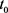$t$ 积分得

$\begin{array}{c}G\left(X\left(t\right)\right)=G\left({c}_{0}\right)+\underset{i=1}{\overset{n}{\sum }}{\int }_{{\alpha }_{i}\left({t}_{0}\right)}^{{\alpha }_{i}\left(t\right)}\left[{f}_{i}\left(s\right)\omega \left({\varphi }^{-1}\left(X\left(s\right)\right)\right)+{g}_{i}\left(s\right)\right]\text{d}s\\ =G\left({c}_{0}\right)+\underset{i=1}{\overset{n}{\sum }}{\int }_{{\alpha }_{i}\left({t}_{0}\right)}^{{\alpha }_{i}\left(t\right)}{g}_{i}\left(s\right)\text{d}s+\underset{i=1}{\overset{n}{\sum }}{\int }_{{\alpha }_{i}\left({t}_{0}\right)}^{{\alpha }_{i}\left(t\right)}{f}_{i}\left(s\right)\omega \left({\varphi }^{-1}\left(X\left(s\right)\right)\right)\text{d}s\end{array}$(14)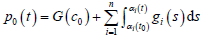(15)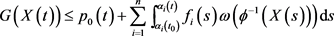(16)

$G\left(X\left(t\right)\right)\le {p}_{0}\left(T\right)+\underset{i=1}{\overset{n}{\sum }}{\int }_{{\alpha }_{i}\left({t}_{0}\right)}^{{\alpha }_{i}\left(t\right)}{f}_{i}\left(s\right)\omega \left({\varphi }^{-1}\left(X\left(s\right)\right)\right)\text{d}s$(17)

(17)式右端为 $Y\left(t\right)$ ，则 $Y\left(t\right)$ 为非减函数， $Y\left({t}_{\text{0}}\right)={p}_{0}\left(T\right)$$X\left(t\right)\le {G}^{-1}\left(Y\left(t\right)\right)$$Y\left(t\right)$$t$ 求导得

$\begin{array}{c}{Y}^{\prime }\left(t\right)=\underset{i=1}{\overset{n}{\sum }}{f}_{i}\left({\alpha }_{i}\left(t\right)\right)\omega \left({\varphi }^{-1}\left(X\left({\alpha }_{i}\left(t\right)\right)\right)\right)\text{\hspace{0.17em}}{{\alpha }^{\prime }}_{i}\left(t\right)\\ \le \omega \left({\varphi }^{-1}\left({G}^{-1}\left(Y\left(t\right)\right)\right)\right)\underset{i=1}{\overset{n}{\sum }}{f}_{i}\left({\alpha }_{i}\left(t\right)\right)\text{\hspace{0.17em}}{{\alpha }^{\prime }}_{i}\left(t\right)\end{array}$(18)

$\frac{{Y}^{\prime }\left(t\right)}{\omega \left({\varphi }^{-1}\left({G}^{-1}\left(Y\left(t\right)\right)\right)\right)}\le \underset{i=1}{\overset{n}{\sum }}{f}_{i}\left({\alpha }_{i}\left(t\right)\right)\text{\hspace{0.17em}}{{\alpha }^{\prime }}_{i}\left(t\right)$(19)

(19)两边对 $t$$t$ 积分，得

$W\left(Y\left(t\right)\right)\le W\left(Y\left({t}_{0}\right)\right)+\underset{i=1}{\overset{n}{\sum }}{\int }_{{\alpha }_{i}\left({t}_{0}\right)}^{{\alpha }_{i}\left(t\right)}{f}_{i}\left(s\right)\text{d}s$

$Y\left(t\right)\le {W}^{-1}\left[W\left({p}_{0}\left(T\right)\right)+\underset{i=1}{\overset{n}{\sum }}{\int }_{{\alpha }_{i}\left({t}_{0}\right)}^{{\alpha }_{i}\left(t\right)}{f}_{i}\left(s\right)\text{d}s\right]$(20)

$u\left(t\right)\le {\varphi }^{-1}\left\{{G}^{-1}\left[{W}^{-1}\left(W\left({p}_{0}\left(T\right)\right)+\underset{i=1}{\overset{n}{\sum }}{\int }_{{\alpha }_{i}\left({t}_{\text{0}}\right)}^{{\alpha }_{i}\left(t\right)}{f}_{i}\left(s\right)\text{d}s\right)\right]\right\},$ (21)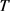的任意性，用 $t$ 代替 $T$ ，即有

$u\left(t\right)\le {\varphi }^{-1}\left\{{G}^{-1}\left[{W}^{-1}\left(W\left({p}_{0}\left(t\right)\right)+\underset{i=1}{\overset{n}{\sum }}{\int }_{{\alpha }_{i}\left({t}_{\text{0}}\right)}^{{\alpha }_{i}\left(t\right)}{f}_{i}\left(s\right)\text{d}s\right)\right]\right\}.$ (22)

${c}_{0}=0$ ，选取小正数 ε > 0 代替 ${c}_{0}$ ，然后让 $\epsilon$ 趋于0，同样证明。

$j=1$ 时， $t\in \left[{t}_{1},{t}_{2}\right)$ ，此时不等式(5)有脉冲项。将(5)变形为

$\begin{array}{c}\varphi \left(u\left(t\right)\right)\le {c}_{0}+\underset{i=1}{\overset{n}{\sum }}{\int }_{{\alpha }_{i}\left({t}_{0}\right)}^{{\alpha }_{i}\left(t\right)}{u}^{q}\left(s\right)\left[{f}_{i}\left(s\right)\omega \left(u\left(s\right)\right)+{g}_{i}\left(s\right)\right]\text{d}s+h\left(t\right){\beta }_{\text{1}}{u}^{m}\left({t}_{\text{1}}-0\right)\\ \le {c}_{0}+\underset{i=1}{\overset{n}{\sum }}{\int }_{{\alpha }_{i}\left({t}_{\text{0}}\right)}^{{\alpha }_{i}\left({t}_{\text{1}}\right)}{u}_{\text{0}}{}^{q}\left(s\right)\left[{f}_{i}\left(s\right)\omega \left({u}_{\text{0}}\left(s\right)\right)+{g}_{i}\left(s\right)\right]\text{d}s+h\left(t\right){\beta }_{\text{1}}{u}_{\text{0}}{}^{m}\left({t}_{\text{1}}-0\right)\\ +\underset{i=1}{\overset{n}{\sum }}{\int }_{{\alpha }_{i}\left({t}_{\text{1}}\right)}^{{\alpha }_{i}\left(t\right)}{u}^{q}\left(s\right)\left[{f}_{i}\left(s\right)\omega \left(u\left(s\right)\right)+{g}_{i}\left(s\right)\right]\text{d}s\\ \le {c}_{1}+\underset{i=1}{\overset{n}{\sum }}{\int }_{{\alpha }_{i}\left({t}_{\text{1}}\right)}^{{\alpha }_{i}\left(t\right)}{u}^{q}\left(s\right)\left[{f}_{i}\left(s\right)\omega \left(u\left(s\right)\right)+{g}_{i}\left(s\right)\right]\text{d}s\end{array}$(23)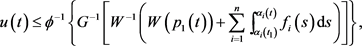(24)(25)

$\begin{array}{c}\varphi \left(u\left(t\right)\right)\le {c}_{0}+\underset{i=1}{\overset{n}{\sum }}{\int }_{{\alpha }_{i}\left({t}_{0}\right)}^{{\alpha }_{i}\left(t\right)}{u}^{q}\left(s\right)\left[{f}_{i}\left(s\right)\omega \left(u\left(s\right)\right)+{g}_{i}\left(s\right)\right]\text{d}s+h\left(t\right)\underset{{t}_{0}<{t}_{j} (26)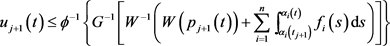(27)

2018年岭南师范学院校级科研项目(理科)重点项目(LZ1806)。

NOTES

*通讯作者。

  Agarwal, R.P. (1982) On an Integral Inequality in n Independent Variables. Journal of Mathematical Analysis and Applications, 85, 192-205. https://doi.org/10.1016/0022-247X(82)90034-8  Agarwal, R.P., Deng, S.F. and Zhang, W.N. (2005) Generaliza-tion of a Retard Gronwall-Like Inequality and Its Applications. Applied Mathematics and Computation, 165, 599-612. https://doi.org/10.1016/j.amc.2004.04.067  Zhang, W.N. and Deng, S.F. (2001) Projected Gronwall-Bellman’s Inequality for Integrable Functions. Mathematical and Computer Modelling, 34, 394-410. https://doi.org/10.1016/S0895-7177(01)00070-X  王五生, 李自尊. 一类乘积形式的非线性时滞积分不等式及其应用[J]. 四川大学学报(自然科学版), 2013, 50(4): 29.  Samoilenko, A.M. and Perestyuk, N.A. (1987) Differential Equations with Impulse Effect. Visha Shkola, Kyiv.  Samoilenko, A.M. and Perestyuk, N.A. (2005) Stability of the Solutions of Differential Equations with Impulsive Action. Applied Mathematics and Computation, 165, 599-605.  Iovane, G. (2007) Some New Integral Inequalities of Bellman-Bihari with Delay for Discontinuous Functions. Nonlinear Analysis, 66, 498-510. https://doi.org/10.1016/j.na.2005.11.043  Gallo, A. and Piccirillo, A.M. (2009) About Some New Analogies of Bell-man-Bihari Type Inequalities Result for Integro-Functions Inequalities with Discontinuous Functions and Their Applications. Nonlinear Analysis, 71, e2276-e2290. https://doi.org/10.1016/j.na.2009.05.019  Gallo, A. and Piccirillo, A.M. (2007) About New Analogies of Gronwall-Bellman-Bihari Type Inequalities for Discontinuous Functions and Estimated Solution for Impulsive Differential Systems. Nonlinear Analysis, 67, 1550-1576. https://doi.org/10.1016/j.na.2006.07.038  Mitropolsky, Y.A., Iovane, G. and Borysenko, S.D. (2007) About a Generalization of Bellman-Bihari Type Inequalities for Discontinuous Functions and Their Applications. Nonlinear Analysis, 66, 2140-2152. https://doi.org/10.1016/j.na.2006.03.006  米玉珍, 钟吉玉. 一类非连续函数的Bellman-Bihari型积分不等式的推广与应用[J]. 四川大学学报(自然科学版), 2015, 49(6): 33-38.